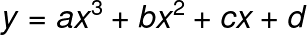# Cubic Regression Calculator

Or input your data as csv

Sharing helps us build more free tools

Fit y = ax³ + bx² + cx + d line using this calculator

You can also perform cubic regression using the quadratic regression calculator by setting order = 3.

## What is Cubic Regression

Cubic regression is used to fit cubic line of best fit to import data. it is very useful when your input data looks like a cubic function.

You can also perform cubic regression using quadratic regression and an order of 3.

## Why Do We Use Cubic Regression

Progression is very useful when the input data looks like a third-order polynomial. We can use cubic regression to find a third order line of best fit to the input data. The cubic regression alcohol or give us the regression coefficients for each Cube x squared x and the constant offset.

## How Many Curves do Cubic Regression Models Have

Cubic regression models typically have two curves. If one or more of the coefficients is very close to 0 then the cubic regression model may appear to have fewer curves.

## Cubic Regression Equation (Cubic Regression Model)

The cubic regression calculator fits a cubic equation to the input dataWhere y is the response variable and x is the predictor variable.

Cubic regression is a special case of multiple linear regression. The line of best fit is a non-linear cubic function. But the cubic regression equation is linear with respect to the coefficients a, b, c, and d.